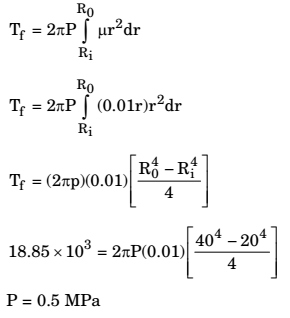## Machine Design Miscellaneous

#### Machine Design

Direction: A 20° full depth involute spur pinion of 4 mm module and 21 teeth is to transmit 15 kW at 960 rpm. Its face width is 25 mm.

1. The tangential force transmitted (in N) is

1. P =15 kW
m = 4 mm
Zp = 21
N = 960 rpm
b = 25mm
Dp =mZp = 4 × 21 = 84 mm

 Speed of pinion, υ = πDpN m / s 60000

 = π × 84 × 960 = 4.22 m / s 60000

Also, P = Ftυ
 or Ft = P = 15 × 1000 υ 4.22

≈ 3552 N

##### Correct Option: A

P =15 kW
m = 4 mm
Zp = 21
N = 960 rpm
b = 25mm
Dp =mZp = 4 × 21 = 84 mm

 Speed of pinion, υ = πDpN m / s 60000

 = π × 84 × 960 = 4.22 m / s 60000

Also, P = Ftυ
 or Ft = P = 15 × 1000 υ 4.22

≈ 3552 N

1. A spur pinion of pitch diameter 50 mm rotates at 200 rad/s and transmits 3 kW power. The pressure angle of the tooth of the pinion is 20°. Assuming that only one pair of the teeth is in contact, the total force (in Newton) exerted by a tooth of the pinion on the tooth on a mating gear is ________.

1. NA

##### Correct Option: A

NA

1. Two mating spur gears have 40 and 120 teeth respectively. The pinion rotates at 1200 rpm and transmits a torque of 20 N.m. The torque transmitted by gear is

1. We know

 Np = TG NG Tp

where,
Np = speed of pinion,
NG = speed of gear wheel
TG = number of teeth of gear,
Tp = number of teeth of pinion
 ∴ 1200 = 120 NG 40

or NG =400 r.p.m.
Since power transmitted by both gear will be equal
i.e. Tpωp = TGωG
where,
Tp = torque transmited by pinion
TG = torque transmitted by gear wheel
 ∴ 20 × 2π × 1200 = TG × 2π × 400 60 60

∴ Torque transmitted by gear, TG = 60 N-m.

##### Correct Option: D

We know

 Np = TG NG Tp

where,
Np = speed of pinion,
NG = speed of gear wheel
TG = number of teeth of gear,
Tp = number of teeth of pinion
 ∴ 1200 = 120 NG 40

or NG =400 r.p.m.
Since power transmitted by both gear will be equal
i.e. Tpωp = TGωG
where,
Tp = torque transmited by pinion
TG = torque transmitted by gear wheel
 ∴ 20 × 2π × 1200 = TG × 2π × 400 60 60

∴ Torque transmitted by gear, TG = 60 N-m.

1. A wire rope is designated as 6 x 19 standard hoisting. The numbers 6 × 19 represent

1. NA

##### Correct Option: C

NA

1. A single-plate clutch has a friction disc with inner and outer radii of 20 mm and 40 mm, respectively. The friction lining in the disc is made in such a way that the coefficient of friction μ varies radially as μ = 0.01 r, where r is in mm. The clutch needs to transmit a friction torque of 18.85 kN mm. As per uniform pressure theory, the pressure (in MPa) on the disc is __________.

1.##### Correct Option: A